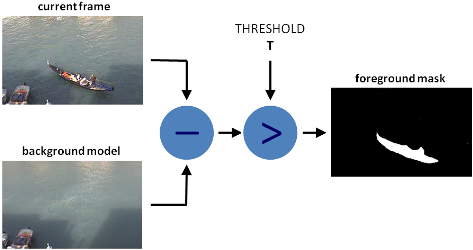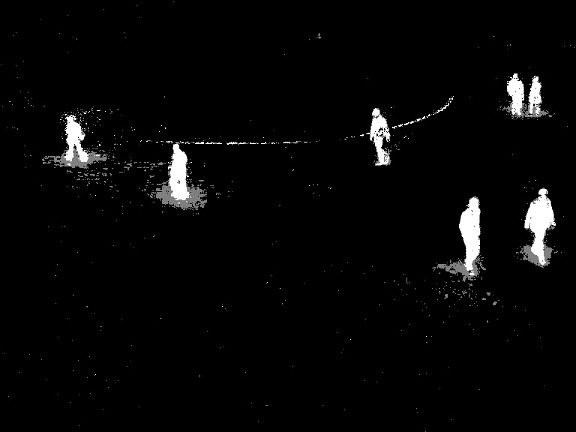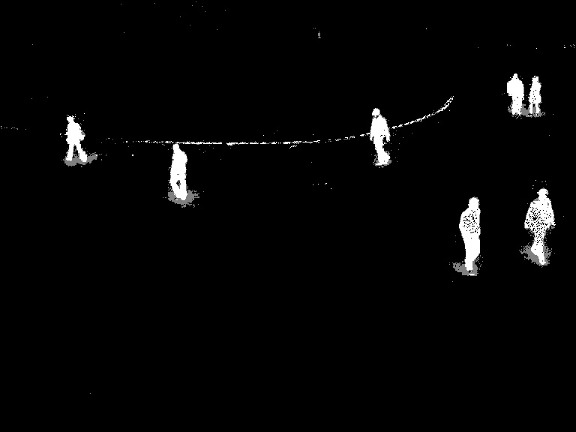OpenCV  4.6.0 Open Source Computer Vision
How to Use Background Subtraction Methods

Prev Tutorial: High level stitching API (Stitcher class)

Next Tutorial: Meanshift and Camshift

Original author Domenico Daniele Bloisi
Compatibility OpenCV >= 3.0
• Background subtraction (BS) is a common and widely used technique for generating a foreground mask (namely, a binary image containing the pixels belonging to moving objects in the scene) by using static cameras.
• As the name suggests, BS calculates the foreground mask performing a subtraction between the current frame and a background model, containing the static part of the scene or, more in general, everything that can be considered as background given the characteristics of the observed scene.• Background modeling consists of two main steps:

1. Background Initialization;
2. Background Update.

In the first step, an initial model of the background is computed, while in the second step that model is updated in order to adapt to possible changes in the scene.

• In this tutorial we will learn how to perform BS by using OpenCV.

## Goals

In this tutorial you will learn how to:

1. Read data from videos or image sequences by using cv::VideoCapture ;
2. Create and update the background model by using cv::BackgroundSubtractor class;
3. Get and show the foreground mask by using cv::imshow ;

### Code

In the following you can find the source code. We will let the user choose to process either a video file or a sequence of images.

We will use cv::BackgroundSubtractorMOG2 in this sample, to generate the foreground mask.

The results as well as the input data are shown on the screen.

## Explanation

We discuss the main parts of the code above:

• A cv::BackgroundSubtractor object will be used to generate the foreground mask. In this example, default parameters are used, but it is also possible to declare specific parameters in the create function.
• A cv::VideoCapture object is used to read the input video or input images sequence.
• Every frame is used both for calculating the foreground mask and for updating the background. If you want to change the learning rate used for updating the background model, it is possible to set a specific learning rate by passing a parameter to the apply method.
• The current frame number can be extracted from the cv::VideoCapture object and stamped in the top left corner of the current frame. A white rectangle is used to highlight the black colored frame number.
• We are ready to show the current input frame and the results.

## Results

• With the vtest.avi video, for the following frame:The output of the program will look as the following for MOG2 method (gray areas are detected shadows):The output of the program will look as the following for the KNN method (gray areas are detected shadows):